Next: Cost Function Up: Nonlinear Factor Analysis Previous: Nonlinear Factor Analysis

## Definition of the Model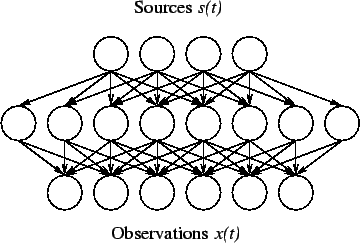The schematic structure of the mapping is shown in Fig. 3. The nonlinearity of each hidden neuron is the hyperbolic tangent, which is the same as the usual logistic sigmoid except for a scaling. The equation defining the mapping is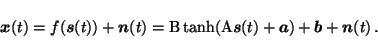(3)

The matrices A and B are the weights of first and second layer and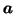and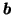are the corresponding biases.

The noise is assumed to be independent and Gaussian and therefore the probability distribution of x(t) is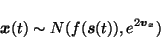(4)

Each component of the vector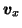gives the log-std of the corresponding component of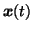.

The sources are assumed to have zero mean Gaussian distributions and again the variances are parametrised by log-std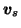.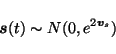(5)

Since the variance of the sources can vary, variance of the weights A on the first layer can be fixed to a constant, which we choose to be one, without loosing any generality from the model. This is not case for the second layer weights. Due to the nonlinearity, the variances of the outputs of the hidden neurons are bounded from above and therefore the variance of the second layer weights cannot be fixed. In order to enable the network to shut off extra hidden neurons, the weights leaving one hidden neuron share the same variance parameter.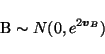(6)

The elements of the matrix B are assumed to have a zero mean Gaussian distribution with individual variances for each column and thus the dimension of the vector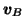is the number of hidden neurons. Both biasesandhave Gaussian distributions parametrised by mean and log-std.

The distributions are summarised in (7)-(12).(7)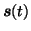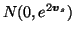(8) AN(0, 1) (9) B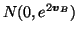(10)N(ma, e2va) (11)N(mb, e2vb) (12)

The distributions of each set of log-std parameters are modelled by Gaussian distributions whose parameters are usually called hyperparameters.N(mvx, e2vvx) (13)N(mvs, e2vvs) (14)N(mvB, e2vvB) (15)

The prior distributions of ma, va, mb, vb and the six hyperparameters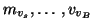are assumed to be Gaussian with zero mean and standard deviation 100, i.e., the priors are assumed to be very flat.Next: Cost Function Up: Nonlinear Factor Analysis Previous: Nonlinear Factor Analysis
Harri Lappalainen
2000-03-03